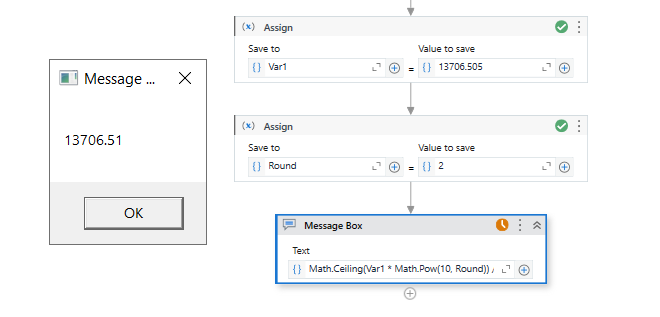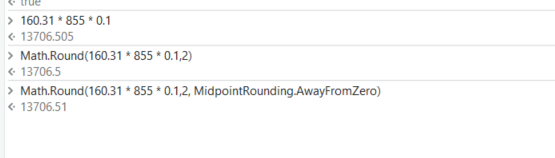# Round off to a decimal number

Hello All,

I am trying to round on one decimal number into calculation. suppose I am multiplying 160.31 x 855 x 0.1= 13706.505, and I want it as 13706.51,
now suppose VarAmount=13706.505, RoundOfLimit=2
I have used math.round(VarAmount, RoundOfflimit) in which RoundOfLimit comes from config file. I have set it to 2. I want round of decimals up to two decimal numbers. Please suggest how to do it.

Assign activity:
VarAmount = 13706.505
RoundOfLimit = 2

Assign activity:
VarAmount = Math.Round(VarAmount, RoundOfLimit)

Variables:
VarAmount (Double) = 13706.505
RoundOffLimit (Int32) = 2

Activities:
Assign:

• To: rounded_amount
• Value: Math.Round(VarAmount, RoundOffLimit)

Write Line:

• Text: rounded_amount.ToString

i tried it but it is giving me 13706.50

``````Assign activity:
VarAmount = 13706.505
RoundOfLimit = 2

Assign activity:
VarAmount = Math.Ceiling(VarAmount * Math.Pow(10, RoundOfLimit)) / Math.Pow(10, RoundOfLimit)

``````
1 Like

this can happen due the internal precision and as we do see the .505 is with the last 5 within the middle and let’s not flip to 51

your code is having only one decimal place, please try it with 3 decimal places. Like 123.325, then it should give as 123.33 in result

but Sir, it is business requirement

@adishjain did you try above one.

it is not a problem. Once we have understood the technical base we can take care of and fullfill the business requirement

1 Like

`Math.Ceiling(Var1 * Math.Pow(10, Round)) / Math.Pow(10, Round)`1 Like
1. Read the value of `VarAmount` from your data source or variable.
2. Read the value of `RoundOfLimit` from your config file or variable. In your case, it’s 2.
3. Use the `Math.Round` method to round `VarAmount` to the specified number of decimal places (`RoundOfLimit`).
VarAmount = Math.Round(VarAmount, RoundOfLimit)
VarAmount = Math.Round(13706.505, 2)

Hope it helps!!

@adishjain Try Above one it will give you desire value

You can try:1 Like

it is giving me 13706.50

yes it is working sir, thankyou so much

it is working for me, thankyou

1 Like